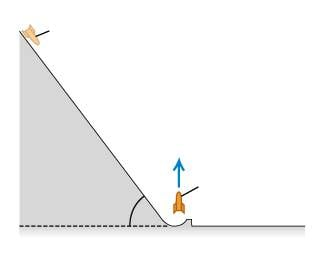# Distance a rocket travels on a launch ramp

[SOLVED] Distance a rocket travels on a launch ramp

## Homework Statement

A 1600kg rocket is to be launched with an initial upward speed of 51.0m/s . In order to assist its engines, the engineers will start it from rest on a ramp that rises 53 degrees above the horizontal. At the bottom, the ramp turns upward and launches the rocket vertically. The engines provide a constant forward thrust of 2000N , and friction with the ramp surface is a constant 500N .How far from the base of the ramp should the rocket start, as measured along the surface of the ramp?

## Homework Equations

K1 + U1 + Wother = K2 + U2

U1 = mgd(sin53)

K1 = 0

Wother = 2000N-500N = (1500N)d

K2 = 1/2m(51m/s)^2

U2 = 0

## The Attempt at a Solution

mgd(sin53) + 1500Nd = 1/2(1600kg)(51m/s)^2

d(mgsin53 + 1500N) = 1/2(1600kg)(51m/s)^2

d = 1/2(1600kg)(51m/s)^2/(mgsin53 + 1500N)

would this be correct?